# Understanding JIT

Published on

The goal of this article is to shed some light on Just In Time compilation. To understand JIT better we will move up from very low level. Our path will run through logic gates, CPU, object code, assembler, linker, and dynamic loader.

### Gates and Circuits

#### Primitive Logic Gates

Basically, a logic gate is a physical implementation of a Boolean function. It has one or more inputs and just one output. Here are few examples: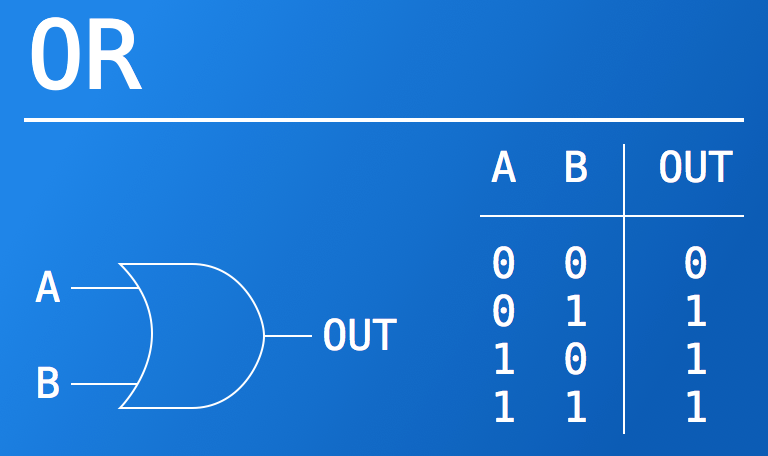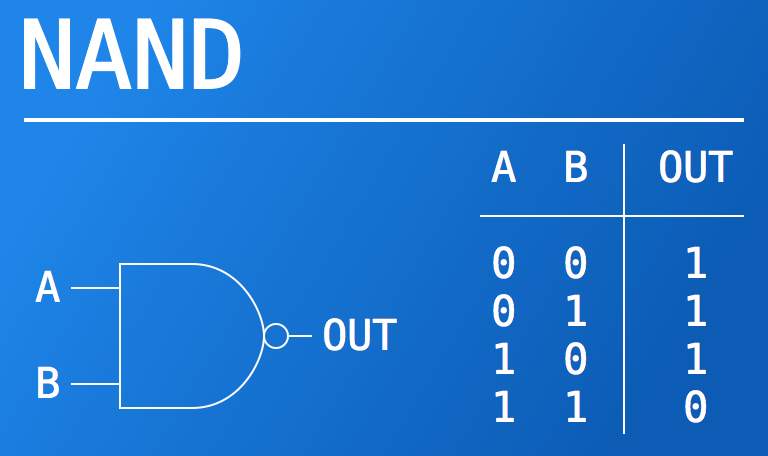#### Complex Logic Gates

Several logic gates can be combined together to form another logic gate.

For example, all primitive logic gates such as `and`, `or`, `not`, etc. can be build from `nand` gates, as presented on the pictures below: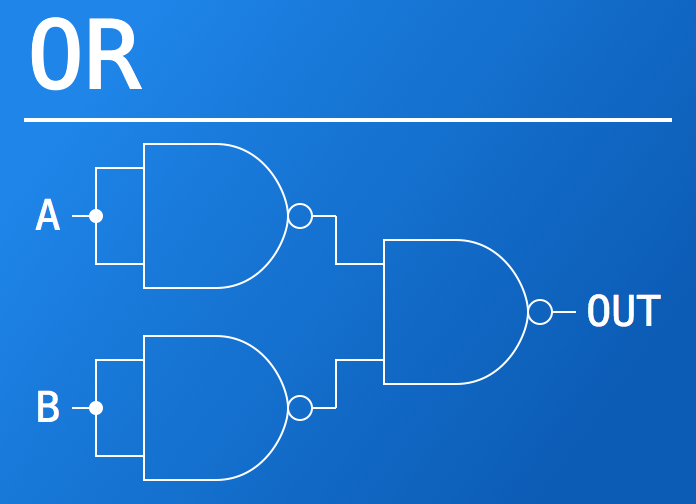#### Logic Circuits

Combination of logic gates can also produce more than just gate, but a logic circuit. These could have more than one output. Example below shows two such circuits.

`Half Adder` takes two binary digits as an input and gives two bits as an output: `sum` holds the sum, while `carry` shows whether ‘overflow’ happened or not.

Nothing describes `Mux` better than this piece of code:

``````int mux(int a, int b, bool sel) {
if (sel) {
return b;
} else {
return a;
}
}``````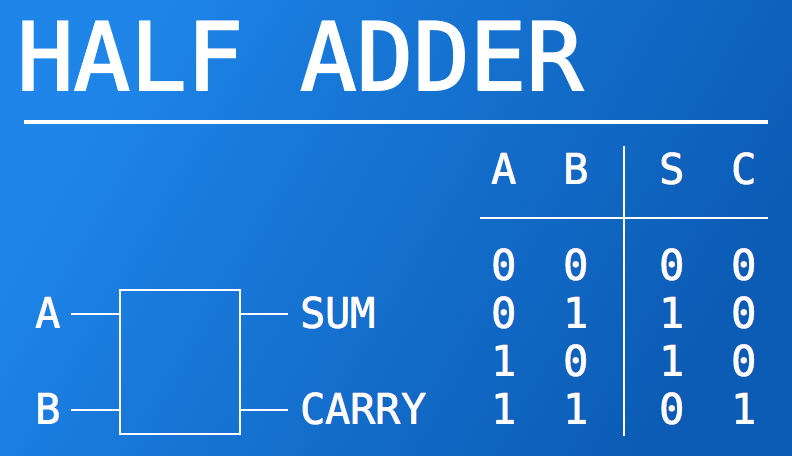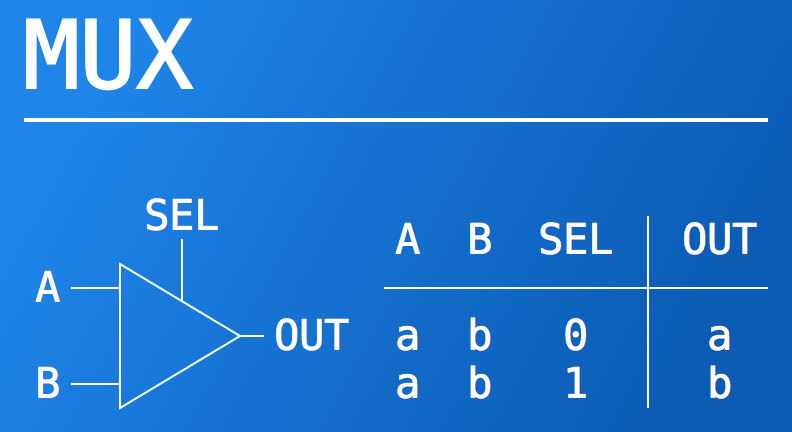### ALU

One can take enough logic gates and circuits and build so-called ALU - Arithmetic Logic Unit.

Here is very simple example just to give you an idea: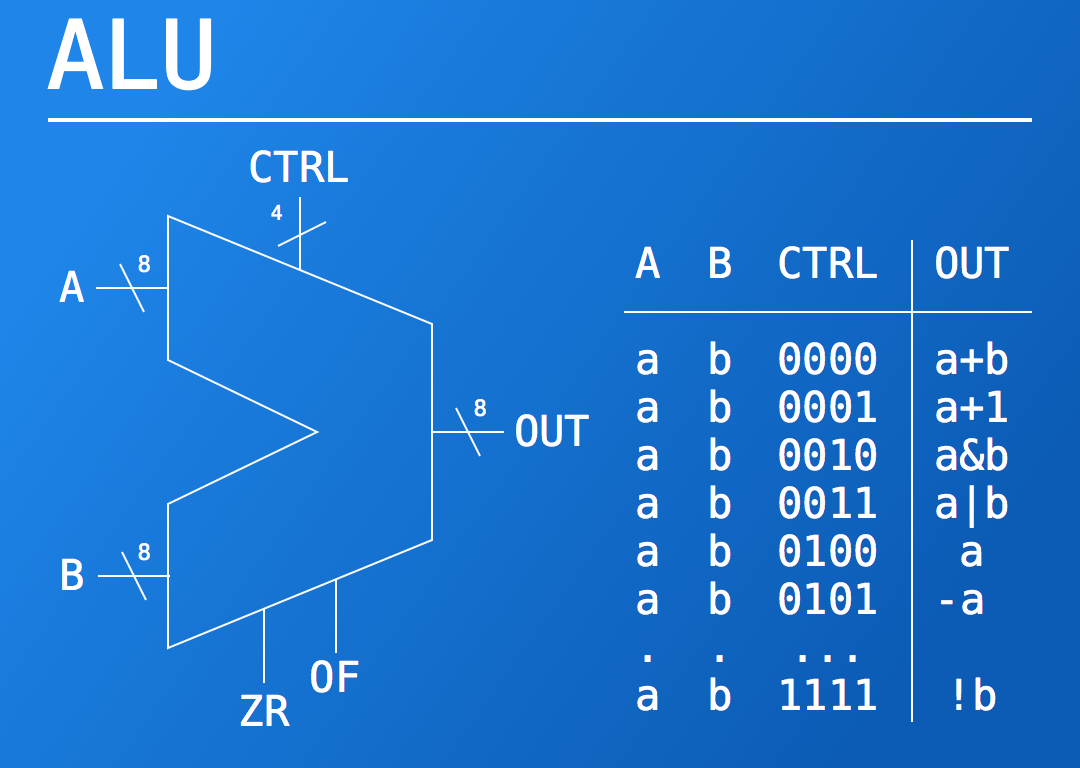ALU is pretty much like `Mux`, but a bit more complex.

It has few components:

• data buses, e.g. two inputs and one output (`A`, `B`, `OUT`)
• opcode or operation code, e.g. control bits on the picture (`CTRL`)
• status, e.g. holds additional information about the result of an operation (`ZR`, `OF`)

Data buses, opcodes and status vary among different ALUs and are defined by an ALU designer.

In the example above data buses have equal width of 8 bits.

The control bits represent different operations, e.g. there are `a+b` and `-b`, but no `a-b` because it’s simply `a+(-b)`. You may find it not efficient, but it’s all about trade-offs: it’s possible to create an ALU which will handle all the operations possible, but such ALU will be more complicated to build, hence higher price and worse characteristics (e.g. higher energy consumption, bigger size, etc.).

The statuses may hold very important information, e.g. indication of whether overflow happened or not. Also, they can be sort of shortcuts: it’s possible to take enough arithmetic operations and determine whether the result is zero, or is negative, or it’s odd or even, but it’s possible to determine it during computation of an operation.

### CPU

ALU is a very important building block of the next circuit - CPU (Central Processing Unit).

Hence, having enough ‘nand’ gates at hand one can build real processor, with several inputs and several outputs:

simple_cpu.png

Having such processor you can take some stream of 0’s and 1’s and, well, process it:

process_zeros_ones.png

For readability you may split them into groups:

``````1101 0011 1100 0111
1001 0101 0110 0000
0001 0110 1001 1010
``````

And this is how processor works: complex logic circuit consumes zeros and ones, and spits zeros and ones as an output.

### Assembly language

Programming a computer using just ones and zeros is extremely hard, non-intuitive, and error-prone. That’s why some smart people came up with a great idea several decades ago. The idea is to use some encoding to represent human-readable zeros and ones with actually human-understandable words, also called mnemonics. For example:

mnemonic_mapping.png

This way one can just write

``````mov ax, bx
ret``````

Which will be converted to actual 0’s and 1’s. This is exactly what happening with assembly or a high level code when it is compiled. These blobs of zeros and ones usually stored on disk for later processing. They referred to as object files. Basically any compiled program on your machine is a big stream of 0’s and 1’s. And some metadata.